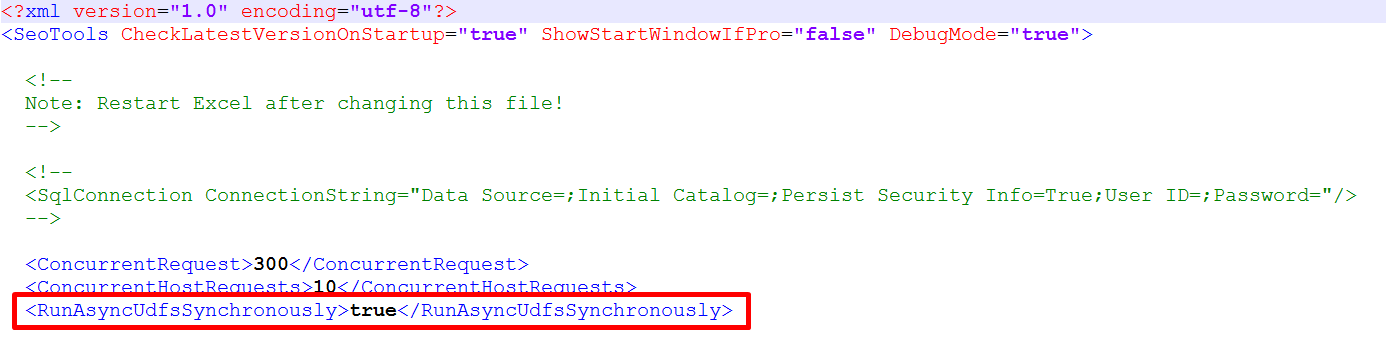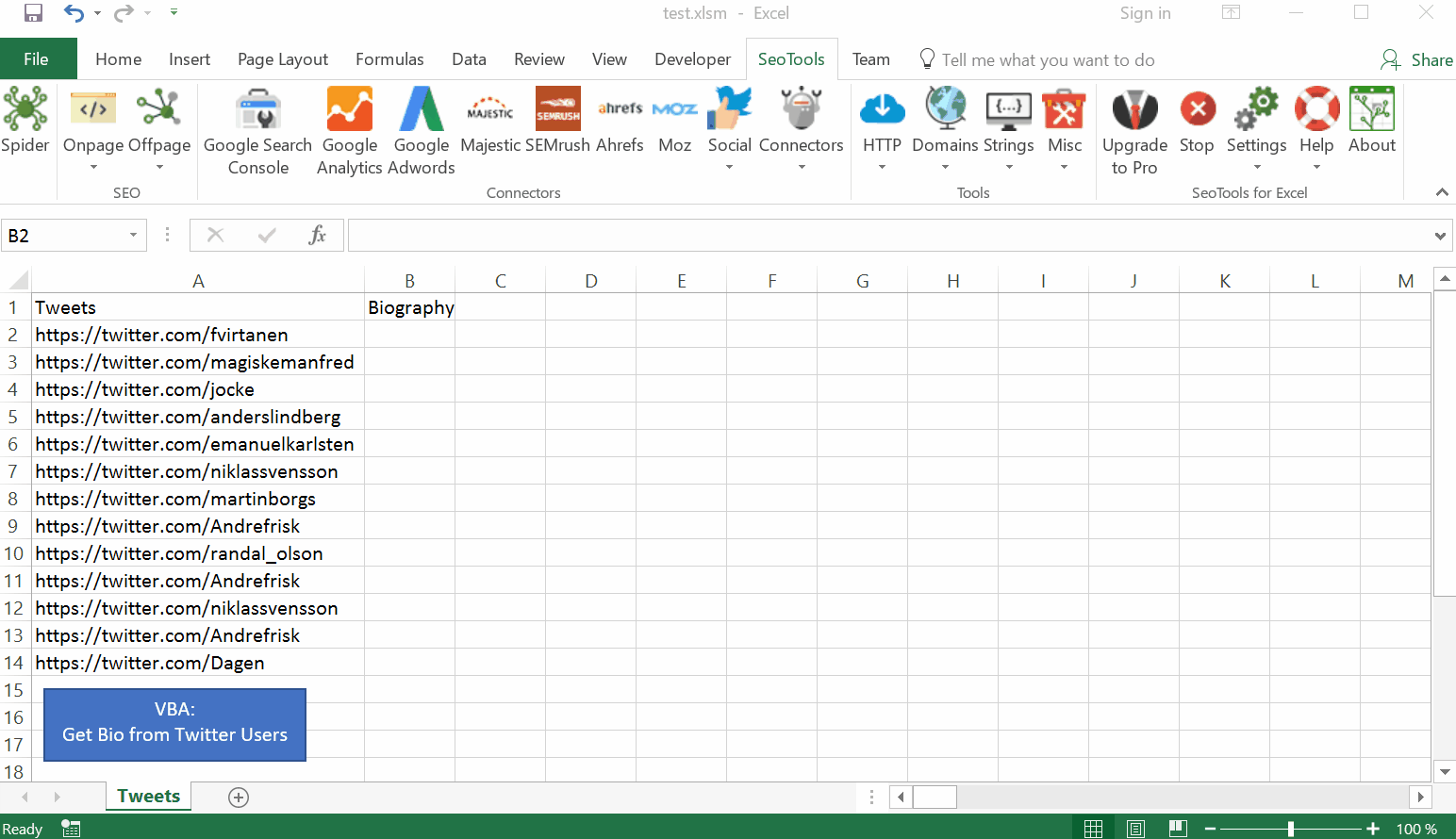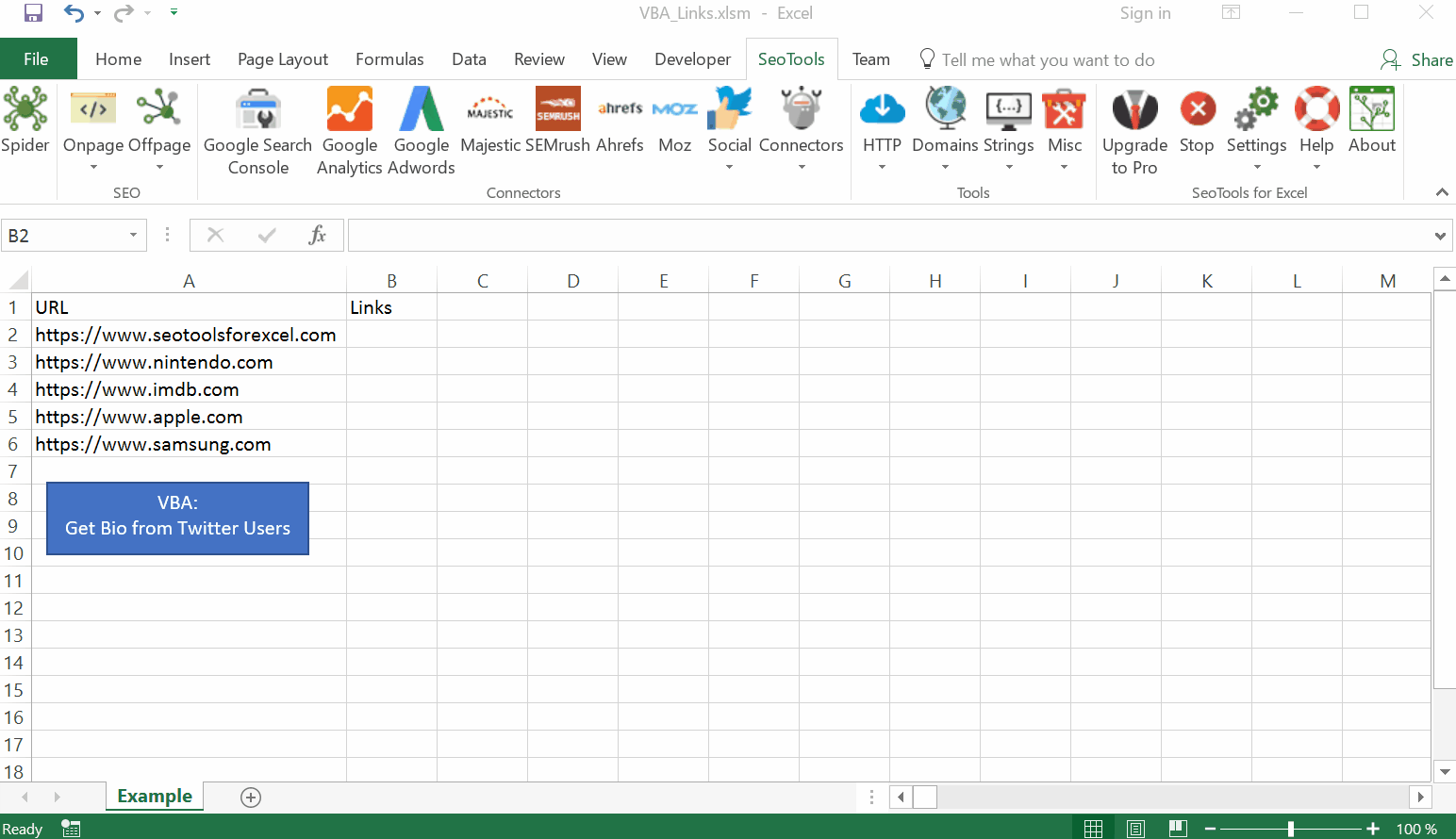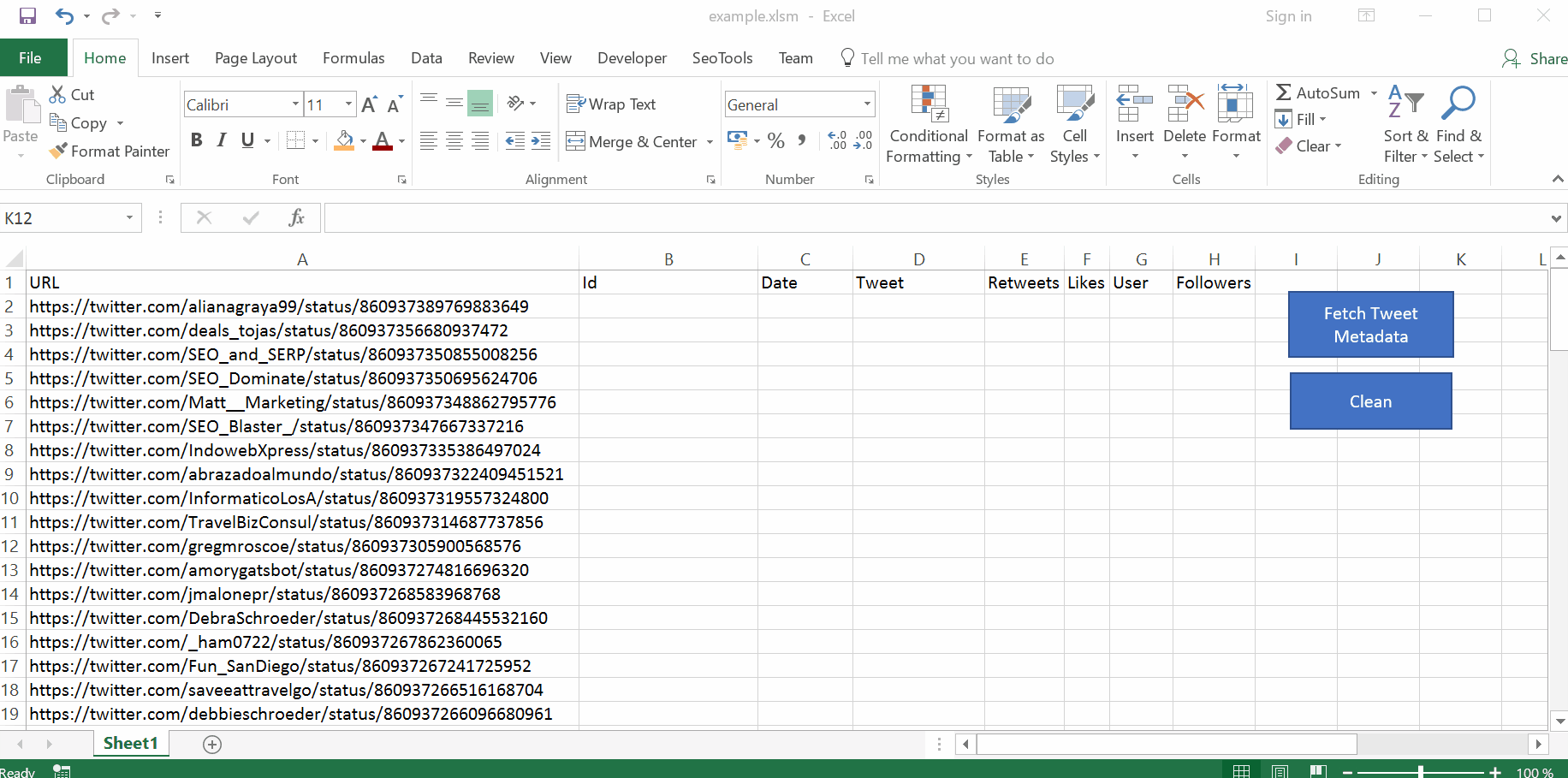Since SeoTools 6.0, most functions run asynchronously. This offers many advantages, such as greatly reduced calculation time and the ability to do other things in Excel while the calculations are running. However, this also means that traditional VBA scripts, which relies on formula based output, will fail. In order to fix this, we recommend two approaches.

## 1. Disable Async

Temporarily make Async functions run synchronously (easier to write VBA but slower)

1. Open SeoTools.Config.Xml

2. Add the following line according to the picture below:
`<RunAsyncUdfsSynchronously>true</RunAsyncUdfsSynchronously>`#### Example Scripts Based on Disable Async``````    Sub GetBio()
Dim row As Integer
Dim col As Integer
row = 2
col = 2
While ActiveSheet.Cells(row, 1) <> ""
ActiveSheet.Cells(row, col) "=XPathOnUrl(A" & row & ", ""//p[@class='ProfileHeaderCard-bio u-dir']"")"
ActiveSheet.Cells(row, col).Copy
ActiveSheet.Cells(row, col).PasteSpecial Paste:=xlPasteValues, Operation:=xlNone, SkipBlanks _
:=False, Transpose:=False
row = row + 1
Wend
End Sub
``````

Extracting an array of all links with CsQueryOnUrl:``````    Sub GetLinks()
Dim row As Integer
Dim col As Integer
row = 2
col = 2
Dim range As Variant
While Sheets("Example").Cells(row, 1) <> ""
Dim xArray() As Variant
xArray =  Evaluate("=TRANSPOSE(CsQueryOnUrl(A" & row & ",  ""a"", ""href""))")
row = row + 1
Wend
End Sub
``````

## 2. Temp Formula

Second option is to output results with formula and then replace results with values when SeoTools fetching is complete. A simple way to know when the fetching is done is to use a Countif formula and count how many cells contain "#GETTING_DATA". When the number reaches zero, it is safe to copy and paste the content as values.

#### Example Scripts Based on Temp FormulaVBA_Tweets.xlsm

``````Sub FetchTweets()
Dim RowCount As Long
RowCount = Range("A" & Rows.Count).End(xlUp).Row
End Sub

Sub Clean()
Columns(2).Copy
Columns(2).PasteSpecial xlPasteValues
End Sub
``````